# piechart

Pie chart

Since R2023b. Recommended over `pie`.

•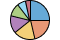## Syntax

``piechart(data)``
``piechart(data,names)``
``piechart(categoricaldata)``
``piechart(tbl,datavar)``
``piechart(tbl,datavar,namesvar)``
``piechart(tbl,categoricalvar)``
``piechart(parent,___)``
``piechart(___,Name=Value)``
``p = piechart(___)``

## Description

### Vector Data

example

````piechart(data)` creates a pie chart of the values in the vector `data`. Each slice has a label indicating its size as a percentage of the whole pie.```

example

````piechart(data,names)` specifies names for the slices. When you specify slice names, they are included in the slice labels.```

example

````piechart(categoricaldata)` creates a pie chart of categorical values. The number of instances of a category determines the size of the corresponding slice, and each slice label includes the category name.```

### Table Data

````piechart(tbl,datavar)` creates a pie chart from the variable `datavar` in the table `tbl`. The resulting pie chart has one slice for each row in the table.```

example

````piechart(tbl,datavar,namesvar)` specifies the variable `namesvar` for the names of the slices. If `namesvar` contains duplicate names, MATLAB® displays multiple slices with the same name.```
````piechart(tbl,categoricalvar)` specifies the categorical variable `categoricalvar`. The number of instances of a category determines the size of the corresponding slice, and each slice label contains the corresponding category name.```

````piechart(parent,___)` creates the pie chart in the specified parent container. The parent container is typically a figure, panel, or tab. Specify `parent` as the first argument in any of the previous syntaxes.```

example

````piechart(___,Name=Value)` specifies properties of the chart using one or more name-value arguments. Specify the name-value arguments after all other input arguments. For example, ```piechart([1 2 3],ExplodedWedges=2)``` creates a pie chart with the second slice offset.For a list of properties, see PieChart Properties.```

example

````p = piechart(___)` returns the `PieChart` object. Use `p` to set properties of the chart after creating it. For a list of properties, see PieChart Properties.```

## Examples

collapse all

Create a pie chart from a vector of numbers. By default, each slice label displays a percentage value.

```data = [1 2 3 4]; piechart(data)```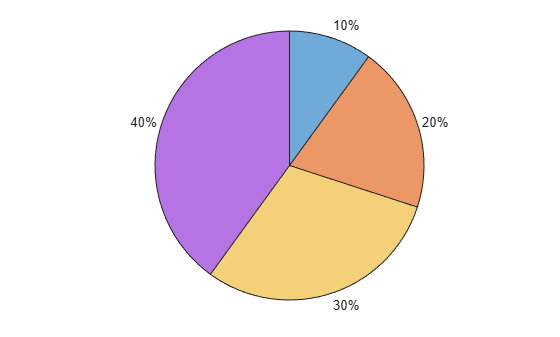Create a pie chart from a categorical vector. The `piechart` function counts the number of instances of each category to determine the size of each slice.

Control the order of the slices by calling the `reordercats` function.

```flavors = categorical(["Banana Cream","Pumpkin","Pumpkin","MATLAB","Blackberry"]); flavors = reordercats(flavors,["MATLAB","Pumpkin","Banana Cream","Blackberry"]); piechart(flavors)```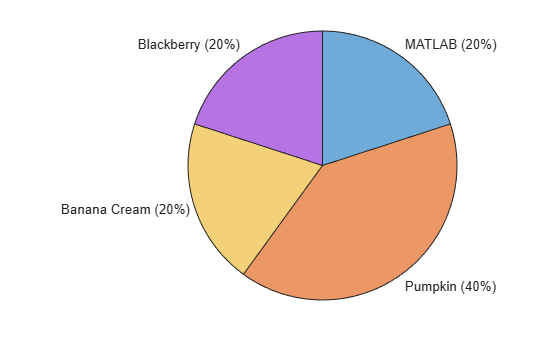Create a table containing the names of bakers in a pie contest and the corresponding number of votes.

```Bakers = ["Betty";"Abby";"Afiq";"Ravi";"Dave"]; Votes = [2; 5; 5; 2; 5]; tbl = table(Bakers,Votes)```
```tbl=5×2 table Bakers Votes _______ _____ "Betty" 2 "Abby" 5 "Afiq" 5 "Ravi" 2 "Dave" 5 ```

Create a pie chart from the table.

`piechart(tbl,"Votes","Bakers")`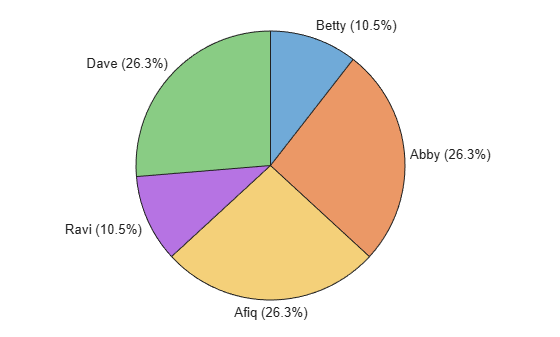You can specify the rotation direction and shift all the slices around the circle by setting properties. You can set properties by specifying name-value arguments when you call the `piechart` function, or you can set properties of the `PieChart` object later.

Create a vector called `data` and use it to create a pie chart. By default, the slices appear in a clockwise direction and the left edge of the first (blue) slice is at `0` degrees (12 o'clock).

```data = [1 2 3 4]; piechart(data)```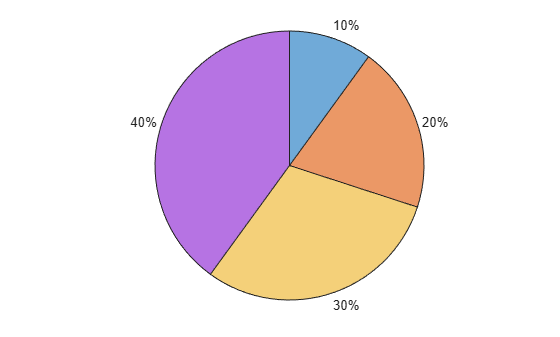Create another pie chart, but this time, set the `Direction` property to `"counterclockwise"` by specifying it as a name-value argument. Call the `piechart` function with an output argument to store the `PieChart` object.

`p = piechart(data,Direction="counterclockwise");`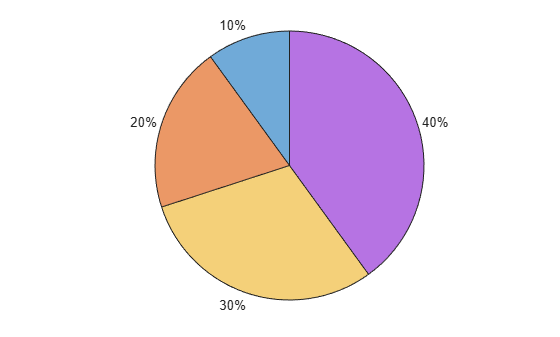Shift the slices by `90` degrees counterclockwise by setting the `StartAngle` property of the `PieChart` object to `-90`.

`p.StartAngle = -90;`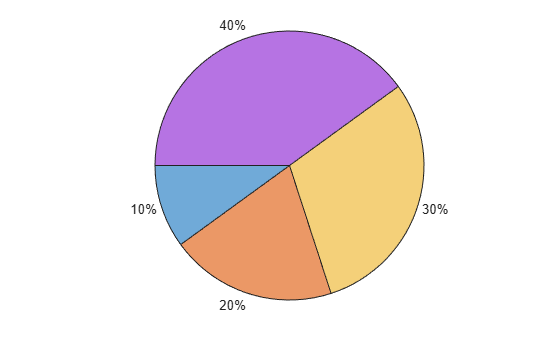You can change the slice labels from percentages to counts by setting the `Labels` property using the numbers from your data vector. If your data is a categorical vector, use the `CategoryCounts` property to get the count values.

Create a pie chart from a numeric vector and a vector of names. Specify an output argument to store the `PieChart` object. Then change the labels to include the data values instead of the percentages.

```data = [1 2 3]; names = ["Blueberry","Pumpkin","Lemon"]; p = piechart(data,names); % Change the labels p.Labels = p.Names + " (" + string(data) + ")";```Now, create a pie chart from categorical data. Change the labels to include the counts of each category instead of the percentages.

```categoricaldata = categorical(["Blueberry","Pumpkin","Pumpkin","Lemon","Lemon","Lemon"]); categoricaldata = reordercats(categoricaldata,["Blueberry","Pumpkin","Lemon"]); p = piechart(categoricaldata); % Change the labels p.Labels = p.Names + " (" + string(p.CategoryCounts) + ")";```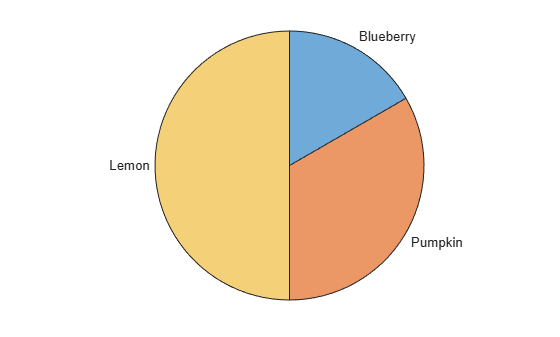Create a pie chart that shows the percentage of total sales for each participant in a bake sale. Call the `piechart` function with an output argument to store the `PieChart` object so you can change aspects of the chart later.

```Bakers = ["Betty","Abby","Afiq","Ravi","Dave"]; Sales = [20 51.55 49.37 20.35 48.25]; p = piechart(Sales,Bakers);```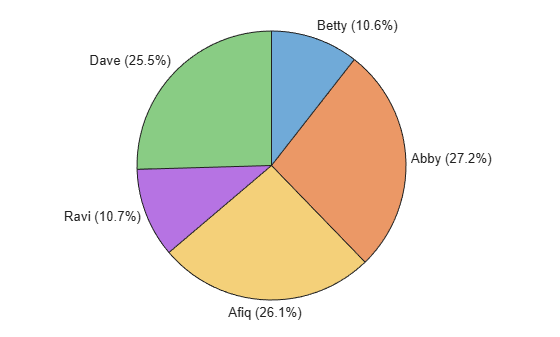Query the slice names of the `PieChart` object.

`p.Names`
```ans = 1x5 string "Betty" "Abby" "Afiq" "Ravi" "Dave" ```

Change the slice names to the pie flavors.

`p.Names = ["Blueberry","Pumpkin","Banana Cream","Blackberry","Key Lime"];`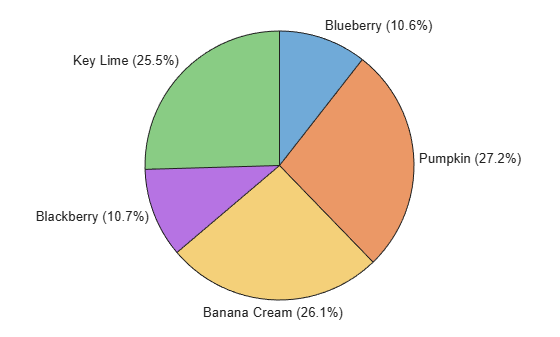Display each label as two lines of text, with the pie flavor on the first line and the sales number on the second line. To combine the different elements for each label, start with the `Names` property, and add a newline character, a dollar sign, and the `Sales` vector (converted to a string).

`p.Labels = p.Names + "\newline\$" + string(Sales);`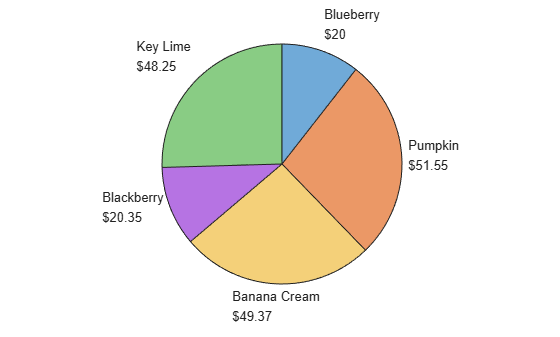You can change the colors of your pie chart by using the `colororder` function. For example, create a pie chart with the default colors.

`piechart([1 2 3 4])`You can choose from several named color palettes, including `gem` (default), `glow`, `sail`, `reef`, `meadow`, `dye`, and `earth`. Change the color palette to `meadow`.

`colororder meadow`Change the color palette to `sail`.

`colororder sail`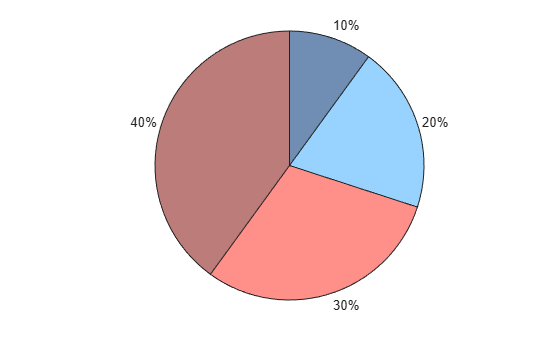To create multiple charts in a figure, use a tiled chart layout.

Create a 1-by-2 tiled chart layout. Create the first chart by calling the `nexttile` function followed by the `piechart` function. Then add a title by calling the `title` function. Repeat these steps for the second chart.

```tiledlayout(1,2) nexttile piechart([1 2 3 4]) title("2022 Totals") nexttile piechart([10 3 1 5 6 4]) title("2023 Totals")```## Input Arguments

collapse all

Slice data, specified as a vector of numeric or duration values. The size of each slice is a percentage of the whole pie, depending on the sum of the elements of `data`:

• If `sum(data)` < `1`, the values of `data` specify the areas of the pie slices, and the result is a partial pie.

• If `sum(data)``1`, MATLAB normalizes the values by `data/sum(data)` to determine the area of each slice of the pie.

Example: `piechart([0.1 0.2 0.3])` creates a partial pie chart.

Example: `piechart([1 2 3 4])` creates a pie chart using values that sum to a number greater than `1`.

Example: `piechart([0.5 0.25 0.25])` creates a pie chart using proportional values that sum to `1`.

Data Types: `single` | `double` | `int8` | `int16` | `int32` | `int64` | `uint8` | `uint16` | `uint32` | `uint64` | `duration`

Slice names, specified as a string vector, cell array of character vectors, or a vector containing numeric, duration, datetime, or categorical values. The vector or cell array must have the same number of elements as `data`. The slice names and the slice percentages are included in the slice labels.

Example: `piechart([1 2 3],["Apples","Cherries","Grapes"])` specifies the names as a string vector.

Example: ```piechart([1 2 3],categorical(["Apples","Cherries","Grapes"]))``` specifies the names as a categorical vector.

Example: `piechart([10 20 30],minutes([1 2 3]))` specifies the names as a duration vector.

Data Types: `single` | `double` | `int8` | `int16` | `int32` | `int64` | `uint8` | `uint16` | `uint32` | `uint64` | `duration` | `datetime` | `categorical` | `string` | `cell`

Categorical slice data, specified as a categorical or logical vector. The size of each slice is proportional to the number of times each value occurs in the vector. The slice names are the category names.

Example: `piechart(categorical(["Apples","Cherries","Grapes","Oranges"]))` creates a pie chart with four slices of the same size.

Example: ```piechart(categorical(["Apples","Cherries","Cherries", "Cherries"]))``` creates a pie chart with a `"Cherries"` slice that occupies 3/4 of the pie. The `"Apples"` slice occupies the remaining 1/4 of the pie.

Data Types: `categorical` | `logical`

Source table containing the slice data, specified as a table or timetable.

Table variable containing the slice data, specified using one of the indexing schemes from the following table. The table variable you specify can contain numeric or duration values.

Indexing SchemeExamples

Variable name:

• A string scalar or character vector.

• A `pattern` object. The pattern object must refer to only one variable.

• `"A"` or `'A'` — A variable named `A`

• `"Var"+digitsPattern(1)` — The variable with the name `"Var"` followed by a single digit

Variable index:

• An index number that refers to the location of a variable in the table.

• A logical vector. Typically, this vector is the same length as the number of variables, but you can omit trailing `0` or `false` values.

• `3` — The third variable from the table

• `[false false true]` — The third variable

Variable type:

• A `vartype` subscript that selects a table variable of a specified type. The subscript must refer to only one variable.

• `vartype("double")` — The variable containing double values

Example: `piechart(tbl,"mydata")` specifies the table variable named `mydata`.

Table variable containing the slice names, specified using one of the indexing schemes from the following table. The table variable you specify can contain a string vector, a cell array of character vectors, or a vector of numeric, datetime, duration, or categorical values.

Indexing SchemeExamples

Variable name:

• A string scalar or character vector.

• A `pattern` object. The pattern object must refer to only one variable.

• `"A"` or `'A'` — A variable named `A`

• `"Var"+digitsPattern(1)` — The variable with the name `"Var"` followed by a single digit

Variable index:

• An index number that refers to the location of a variable in the table.

• A logical vector. Typically, this vector is the same length as the number of variables, but you can omit trailing `0` or `false` values.

• `3` — The third variable from the table

• `[false false true]` — The third variable

Variable type:

• A `vartype` subscript that selects a table variable of a specified type. The subscript must refer to only one variable.

• `vartype("double")` — The variable containing double values

Example: `piechart(tbl,"mydata","mynames")` creates a pie chart using the slice data in the variable `mydata` and the slice names in the variable `mynames`.

Table variable containing the categorical data, specified using one of the indexing schemes from the following table. The table variable you specify can contain categorical data or logical values. The number of instances of a category determines the size of the corresponding slice.

Indexing SchemeExamples

Variable name:

• A string scalar or character vector.

• A `pattern` object. The pattern object must refer to only one variable.

• `"A"` or `'A'` — A variable named `A`

• `"Var"+digitsPattern(1)` — The variable with the name `"Var"` followed by a single digit

Variable index:

• An index number that refers to the location of a variable in the table.

• A logical vector. Typically, this vector is the same length as the number of variables, but you can omit trailing `0` or `false` values.

• `3` — The third variable from the table

• `[false false true]` — The third variable

Variable type:

• A `vartype` subscript that selects a table variable of a specified type. The subscript must refer to only one variable.

• `vartype("double")` — The variable containing double values

Example: `piechart(tbl,"mycats")` specifies the table variable named `mycats`.

Parent container, specified as a `Figure`, `Panel`, `Tab`, `TiledChartLayout`, or `GridLayout` object.

### Name-Value Arguments

Specify optional pairs of arguments as `Name1=Value1,...,NameN=ValueN`, where `Name` is the argument name and `Value` is the corresponding value. Name-value arguments must appear after other arguments, but the order of the pairs does not matter.

Example: `piechart([1 2 3 4],StartAngle=90)` creates a pie chart with a starting angle of `90` degrees.

Note

The properties listed here are only a subset. For a full list, see PieChart Properties.

Offset slices, specified as a numeric or logical vector for numeric data. If you create the chart using categorical data, you can specify a string vector or a character vector containing one or more category names. The orange slice in this pie chart is offset.Example: `piechart([5 7 4 6],ExplodedWedges=3)` creates a pie chart with the third slice offset.

Example: `piechart([5 7 4 6],ExplodedWedges=[1 3])` creates a pie chart with the first and third slice offset.

Example: `piechart([5 7 4 6],ExplodedWedges=[false false true false])` creates a pie chart with the third slice offset.

Example: `piechart(categorical(["A" "B" "C" "D"]),ExplodedWedges="B")` creates a pie chart using categorical data with slice `B` offset.

Starting angle of the first slice, specified as a scalar value in degrees. By default, the starting angle is `0` degrees. Positive values rotate the slices in a clockwise direction. Negative values rotate the slices in a counterclockwise direction.

You can envision the location of the starting angle by considering the arrangement of numbers on a clock. A starting angle of `0` degrees corresponds to 12 o'clock, and a starting angle of `90` degrees corresponds to 3 o'clock.Data Types: `single` | `double` | `int8` | `int16` | `int32` | `int64` | `uint8` | `uint16` | `uint32` | `uint64`

Direction for adding slices, specified as `"clockwise"` or `"counterclockwise"`.

• `"clockwise"` — Add slices in a clockwise direction.

• `"counterclockwise"` — Add slices in a counterclockwise direction.

## Output Arguments

collapse all

`PieChart` object, which is a standalone visualization. Use `p` to set properties on the pie chart after creating it.

collapse all

### Standalone Visualization

A standalone visualization is a chart designed for a special purpose that works independently from other charts. Unlike other charts such as `plot` and `surf`, a standalone visualization has a preconfigured axes object built into it, and some customizations are not available. A standalone visualization also has these characteristics:

• It cannot be combined with other graphics elements, such as lines, patches, or surfaces. Thus, the `hold` command is not supported.

• The `gca` function can return the chart object as the current axes.

• You can pass the chart object to many MATLAB functions that accept an axes object as an input argument. For example, you can pass the chart object to the `title` function.

## Version History

Introduced in R2023b